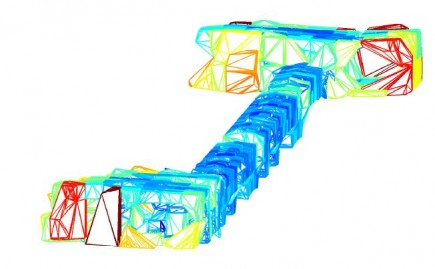# Computational Geometry

The field of computational geometry is focused on finding efficient algorithms for problems that have a geometrical basis. Geometrical algorithms form the basis for the Geometry and Motion planning department and hence it is one area where we have particular strength. At FCC, we are constantly developing cutting edge algorithms to push the limit of what was thought possible within computational geometry.

## Details

Currently all the activities in the Geometry and Motion planning department are based, in some form, on efficient algorithms for geometric problems. We develop and have high competencies in numerous areas such as:

• Space partitioning tools, e.g. bounding volume hierarchies, kd-trees and octrees.
• Mesh operations, e.g. simplifications, merging and measuring.
• Optimization, e.g. linear programming, genetic algorithms and tabu searches.
• Geometric operations, e.g. convex hull and maximum volume computations.
• Graph based algorithms, e.g. shortest distance algorithms and max flow-min cut.The convex hull of numerous objects in different colors.

## Application Areas

The main applications of such tools at FCC are to robot motion planning, computer graphics, querying geometric objects, rigid body path planning as well as to our core base of algorithms powering just about everything we do in the Geometry and Motion planning department.

© 2014-2022  Fraunhofer-Chalmers Centre